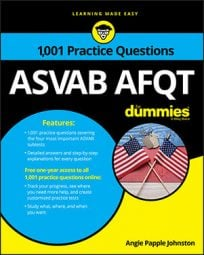##### ASVAB AFQTThe Mathematics Knowledge subtest on the ASVAB will throw one or two problems at you that involve systems of equations. As you'll see in the following practice questions, they may look a little difficult, but you can solve them using either the elimination or substitution method.

## Practice questions

1. Solve:

A. (3, 4) B. (4, 3) C. (–3, 4) D. (–3, –4)

2. Solve the system:

A. x = 5 and y = 1 B. x = 4 and y = 1 C. x = 4 and y = 2 D. x = 5 and y = 2

1. The correct answer is Choice (C).

Solve this system by using the elimination method. Multiply each factor in the second equation by –1 and then add the equations:

The solution is –2y = –8, which you can solve normally:

Put y into the simplest equation (although either equation will work) to solve for x:

2. The correct answer is Choice (B).

When you solve a system, it's given that x is the same in both equations; so is y.

One approach is to isolate one variable in one equation by solving xy = 3:

Replace x in the first equation with 3 + y:

Because y = 1, you can substitute 1 for y in the original equation to find x: